# Algorithm, complexity of description of an

program-size complexity

A measure which characterizes the length of description of an algorithm. The complexity of description of an algorithm is defined differently, depending on the specific definition. At present (1987) there is as yet no generally valid definition of the concept, and the most frequently occurring cases are reviewed below.

The complexity of description of a normal algorithm is usually understood to mean the length of its encoding, i.e. the length of all its substitution formulas when written in one line (a special separating character is interposed between the individual formulas). The complexity of description of a Turing machine usually means the number of its internal states and external symbols. A Turing machine may also be characterized by the number of commands of the machine in question. In the case of recursive functions (cf. Recursive function) given by recursion schemes, the number of letters in these schemes usually serves as the measure of their complexity.

An axiomatic definition of the complexity of description of an algorithm has been proposed . Below the application of this definition to Turing machines will be discussed. Let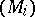,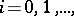be the natural numbering of Turing machines characterized by the fact that the machine itself (i.e. its program) may be effectively reconstituted from its number, and the number of the machine may be effectively reconstituted from the machine (i.e. from its program). A general recursive functionis a measure of the complexity of the machines (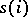being the complexity of the machine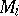) if and only if: a) for anythere exists not more than a finite number of machines with complexity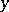; and b) there exists an effective procedure which makes it possible to determine, for any, all the machines with complexity.

Letbe an arbitrary measure of the complexity of a Turing machine. Ifis an arbitrary, effectively (i.e. algorithmically) enumerable infinite subclass of Turing machines, then there exists a machinebelonging to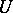, and there exists a machine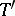(which may or may not belong to) such thatandcompute the same function, and the complexity ofis much lower than that of. Hence it follows, in particular, that there exist primitive recursive functions whose lowest complexity in the primitive recursive form (i.e. through schemes of primitive recursion) is much higher than their lowest complexity in the general recursive form (i.e. through schemes of general recursion). Let the complexity of normal algorithms and of Turing machines mean, respectively, the length of the encoding and the number of internal states. Then it will be possible to realize any function of the algebra of logic in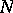variables (cf. Boolean function) : a) by a normal algorithm in an-letter alphabet with complexity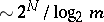; and b) by a Turing machine with an-letter external alphabet with complexity. The study of the complexity of algorithms solving the finite restrictions of algorithmically unsolvable problems (the so-called bounded algorithmic problems) began in the 1960s. A.A. Markov considered the following problem: To construct, for any function of the algebra of logic invariables, an encoding of a normal algorithm in the alphabet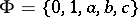which realizes the function and which has least complexity among all such algorithms. It has been shown ,  that the complexity of a normal algorithm which solves this problem is of the order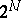. Another problem which has been studied is the complexity of algorithms which solve, for the firstnatural numbers, the problem of belonging to a recursively enumerable set (the complexity of-segments of recursively enumerable sets). It was shown that in the case of normal algorithms, the order of such a complexity is not more than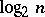, and that this estimate cannot be reduced in the general case. There also exist sets defined by simple diagonalization of which the-segments have complexity order. It was also shown that if the time of operation of algorithms is general-recursively bounded, then the complexity of-segments of recursively enumerable sets may increase exponentially, and the exponent may attain value.

Complexity of description of an algorithm is used in formalizations of the problem of the minimum complexity of an algorithm which constructs some finite object. This minimum complexity is often named simply the complexity of the finite object (for this particular specification of the complexity of description of an algorithm).

A definition of the complexity of finite objects was first proposed by A.N. Kolmogorov (cf. Algorithmic information theory). It was found that the following asymptotically exact relations exist between the complexityaccording to Kolmogorov, the complexity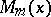of the same objects when expressed through the length of the encoding of a normal algorithm into an-letter alphabet, and the complexity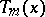expressed by the number of internal states of a Turing machine with an external-letter alphabet: